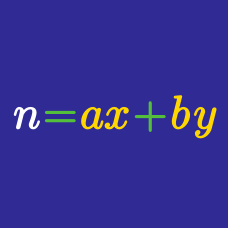Number Theory

Integer Equations - Stars and Bars

A vote to elect the class president is being held in a classroom with $8$ students. There are $3$ nominees, who do not get to vote. If the vote is a secret vote, i.e. the people do not know who voted for whom, what is the number of possible vote count results?

How many ordered sets of non-negative integers $(a, b, c, d)$ are there such that $a + b +c+d =13$?

Consider the two sets $A=\{1,2,3,4\}$ and $B=\{5,6,7,8\}.$ How many possible functions $f:A\rightarrow B$ are there such that if $i then $f(i)\le f(j)?$

Calvin took a trip to the store to purchase some lightbulbs. His choices at the store were $25W, 40W, 60W, \mbox{ and } 100W$ lightbulbs. If Calvin purchased $13$ lightbulbs, how many different combinations of bulbs could he have purchased?

A vote to elect the class president is being held in a classroom with $10$ students. There are $3$ nominees, who do not get to vote. If the vote is a secret vote, i.e. the people do not know who voted for whom, what is the number of possible vote count results?

×